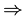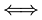# Implication and Iff

The Symbols areand## Implication:Implication says "if ... then"

### Example:

If both a and b are odd numbers then (a+b) is even

can be written as:

both a and b are odd numbers(a+b) is even

The reason it points to the right is that it might not be true the other way

### Example:

Just because (a+b) is even does not mean that a and b are odd (they may both be even)

## Iff:Iff says "if and only if"

It is an implication that goes both ways.

### Example:

x + y = 3x = 3 − y

That one is true both ways!

### Example:

a and b are both integers.

If one of a and b is odd and the other is even then (a+b) is odd can be written as:

One of a and b is odd and the other is even(a+b) is odd

In this case it goes both ways, because if (a+b) is odd and a and b are both integers, then one of them must be odd and the other even.